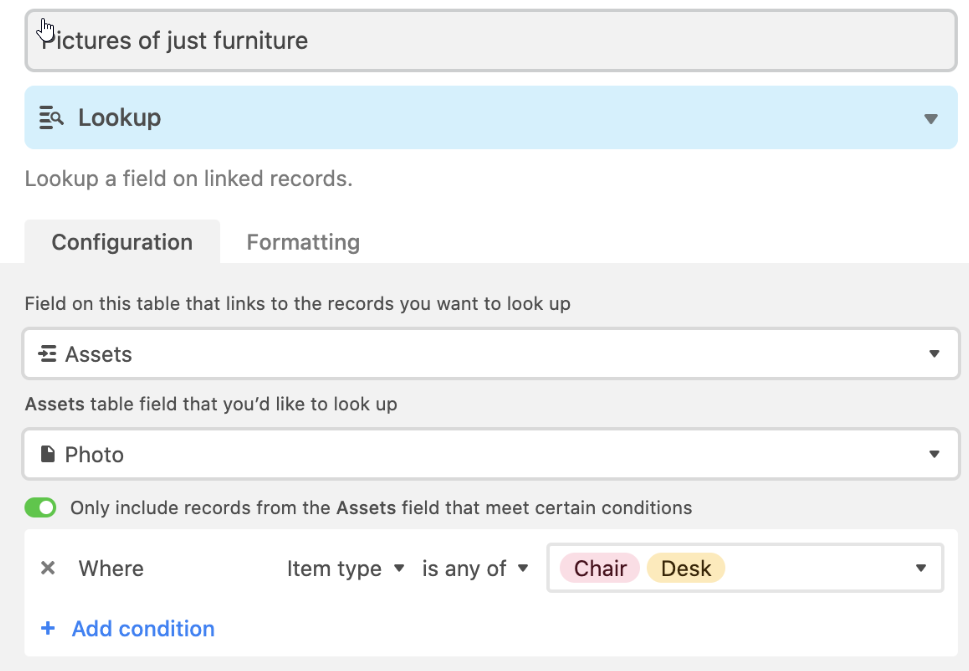# LookUp with conditions

This would make it possible to retrieve values from another table with conditions.
Here an example:2 Likes

We’ll discuss the suggestion on the team call, thanks for your activity @moondayAs soon as I have some updates on your requests, I’ll post them here.

1 Like

@moonday For now you can use the `filter` formula function to achieve the same effect. For example you could create a formula field with the formula:

``````filter(
lookup('Assets in Room', 'Price'),
or(
totext(lookup('Assets in Room', 'Asset Type')) = "Chair",
totext(lookup('Assets in Room', 'Asset Type')) = "Desk")
)
``````

Its more complex unfortunately than the filter builder method suggested which we do want to add, but hopefully helps for now. I also invited you to a Baserow workspace I made showing the above formula in action.

One advantage of the formula way is you can then aggregate the results like so:

``````sum(filter(
lookup('Assets in Room', 'Price'),
or(
totext(lookup('Assets in Room', 'Asset Type')) = "Chair",
totext(lookup('Assets in Room', 'Asset Type')) = "Desk")
))
``````
3 Likes

Hi @nigel , thank you very much for sharing this.
& yes, this looks a little “big” & not that ux-friendly - but anyway, for now it works.

Hey @moonday! Some updates on your request: we do plan to work on this feature, but it’s not a priority one. For now, you need to use formulas to set up conditions in the lookup as Nigel suggestedHi @olgatrykush sure,
As there is a workaround totally understandable.
Maybe you should think of a way to make this solution option more prominent - as it will eventually drop from the easy accessible list of things here in the community. Maybe a dedicated Formula page + this as an addon - info?

Hi @nigel! I’m also interested in your formulascould you invite me to your workspace so that I can have a look to your exemple? Thanks